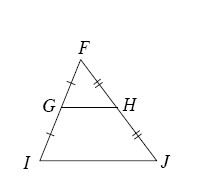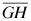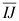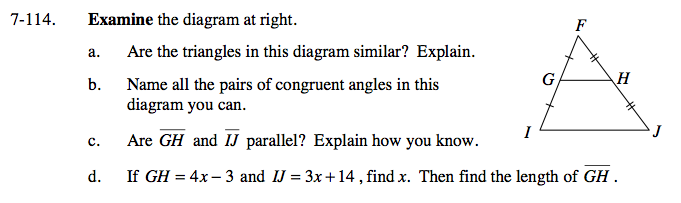### Home > GC > Chapter 7 > Lesson 7.3.2 > Problem7-114

7-114.
1. the diagram below. Homework Help ✎1. Are the triangles in this diagram similar? Explain.

2. Name all the pairs of congruent angles in this diagram you can.

3. Areandparallel? Explain how you know.

4. If GH = 4x − 3 and IJ = 3x + 14 , find x. Then find the length of.Do the marks help prove they are similar? If so, how? What makes these triangles similar? Are there any angles that are congruent?

Yes, by SAS~ because Segment FI is proportional to segment FG, ∠F ≅ ∠F, and segment FJ is proportional to segment FH.

Look at the triangles, what angles are corresponding to each other?

How can knowing sets of congruent angles, help you prove the sides are parallel?

Yes they are parallel, because corresponding angles are congruent.

$\text{How are the lengths of}\ \overline{GH }\; \text{and} \; \overline{ IJ }\; \text{related? How will this help you solve for}\ x?$